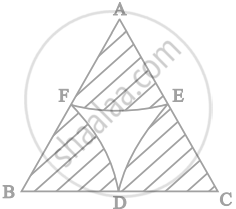# In figure, arcs are drawn by taking vertices A, B and C of an equilateral triangle of side 10 cm. to intersect the sides BC, CA and AB at their respective mid-points D, E and F. Find the area of the - Mathematics

Sum

In figure, arcs are drawn by taking vertices A, B and C of an equilateral triangle of side 10 cm. to intersect the sides BC, CA and AB at their respective mid-points D, E and F. Find the area of the shaded region (Use π = 3.14).#### Solution

ABC is an equilateral triangle.

And, AB = BC = CA = 10 cm

As, D, E, F are mid-points of the sides,

AE = EC = CD = BD = BF = FA = 5 cm

∠A = ∠B = ∠C = 60°

Area of sector CDE = theta/360 xx pir^2

= 60/360 xx pi (5)^2

= 13.0833 cm2

Area of shaded region = 3 × Area of sector CDE

= 3× 13.0833

= 39.25 cm2

Concept: Areas of Combinations of Plane Figures
Is there an error in this question or solution?

#### APPEARS IN

NCERT Mathematics Exemplar Class 10
Chapter 11 Area Related To Circles
Exercise 11.3 | Q 12 | Page 127
Share